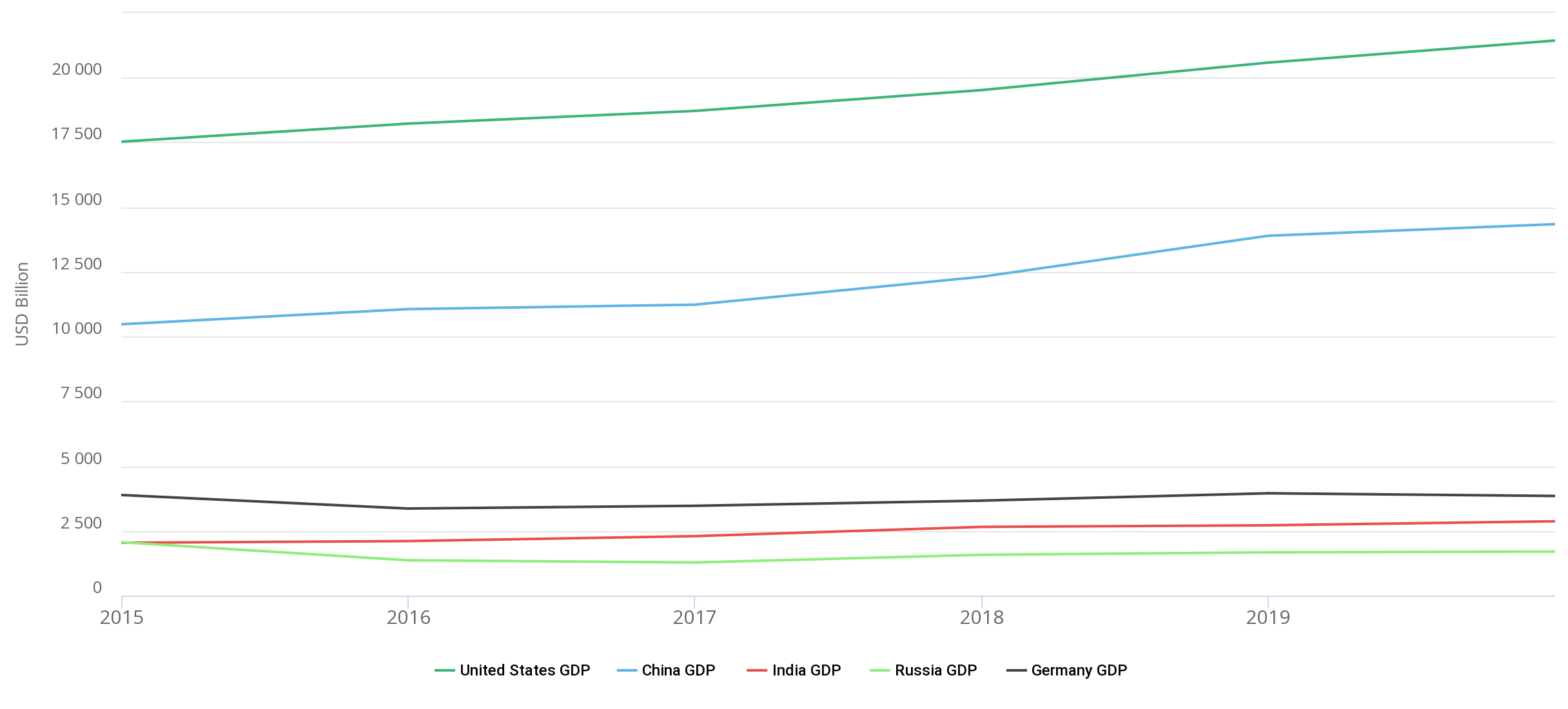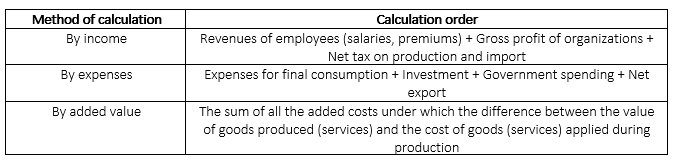Hint mode is switched on
Glossary

# GDP

(Gross domestic product) is a macroeconomic indicator that shows the total market value of all finite goods and services produced in the state for a certain period.

GDP is an indicator that is used to assess economic growth in the country, as well as to compare the level of development of the economy with other countries.

GDP is most often calculated by national statistical offices and international institutions. For example, in Germany, this is the Federal Statistical Office. In addition, GDP can be counted and published by central banks, such as the Central Bank of Costa Rica. GDP is usually evaluated in national currency or in the world currency (US dollar). International Institutions (the World Bank, IMF, and others) consolidate national data and recalculate them into the US dollar.

The graph below shows the dynamics of GDP in billions of US dollars for countries from different regions of the world over the past few years, according to the World Bank.The table presents the main methods for calculating GDP.As examples of calculating GDP for added value, it is possible to provide GDP indices calculated in various sectors of the economy (in transport, in construction, in the services sector). Thanks to these data, it is possible to understand the importance of industries for the economy and find out which countries are agricultural (most of the GDP is formed in agriculture), industrial (most of the GDP is formed by industry), or post-industrial (services occupy a major role in the production of added value).

In macroeconomic analysis, dynamics of gross domestic product can be tracked and predicted, and calculation of the annual growth rate of GDP could be made (for example, in Russia, Greece, Denmark, Finland, Albania). The dynamic means the growth rate of the economy. “Economy” can be used instead of the word "GDP."

Most governments are aimed at the growth of their economy and conduct monetary and financial policies that, in their opinion, will better contribute to this. For example, 1) reduce taxes, which will contribute to the growth of income of citizens and enterprises and contribute to an increase in their costs. 2) Increase government spending that, with other things being equal, gives an increase in expenditure GDP and increase public revenues of public funds. 3) Reduce the interest rate to stimulate investment growth and consumption.

In addition to analyzing the dynamics of GDP, the structure of GDP for each method and the dynamics of changes in the structure are analyzed.

To assess the growth of the economy, you need to understand that prices are constantly changing. It brings us to two concepts: nominal GDP and real GDP. Nominal GDP is calculated in current market prices and depends on the price level in the economy. For example, if prices are growing, that is, inflation in the country, then nominal GDP will also increase with constant production volumes.

By real GDP, in turn, the GDP in constant prices is understood - that is, an indicator that is measured in the prices of the base year with which the comparison is made. The base year statistics authority determines independently. Real GDP is calculated as the ratio of nominal GDP to the price index. In this case, the price index represents the percentage of prices of this year to the prices of the base year (which is called the GDP deflator).

When they say that “GDP grew/fell by n%,” they give a real estimate, i.e., minus inflation. Some people mistakenly begin to subtract consumer inflation from this figure, which leads to completely incorrect data.

Examples of GDP in constant prices: Russia, USA, Germany, Vietnam, India, Mexico, Serbia, etc.). The following are examples of GDP at current prices: Kazakhstan, Uzbekistan, Armenia, China, and Spain.

GDP can be calculated on an annual (for example, in Bangladesh), quarterly (in Argentina), and monthly basis (for example, in Azerbaijan). Most countries provide quarterly estimates, so 4 quarters need to be added to calculate annual GDP. And for monthly data, add up the 12 months of the year. The United States stands out as it publishes its GDP estimate on a quarterly basis but gives it in a seasonally adjusted annual rate (SAAR). Roughly speaking, the conditions of the quarter are multiplied by 4.

Using the data on GDP and the country’s population, you can calculate the GDP per capita. This indicator is equal to the ratio of GDP to the average annual number of state residents and shows the level of well-being of the population. Usually, the higher this indicator, the more income people have.

In addition, there is such an indicator as a gross national product (GNP). Its main difference from GDP is that the GNP takes into account the benefits produced by its residents both in the country itself and beyond its borders, while GDP takes into account the benefits produced only within the country. GNP is calculated, for example, in Canada, and in practice, it is used less frequently than the GDP indicator.
Registration is required to get access.